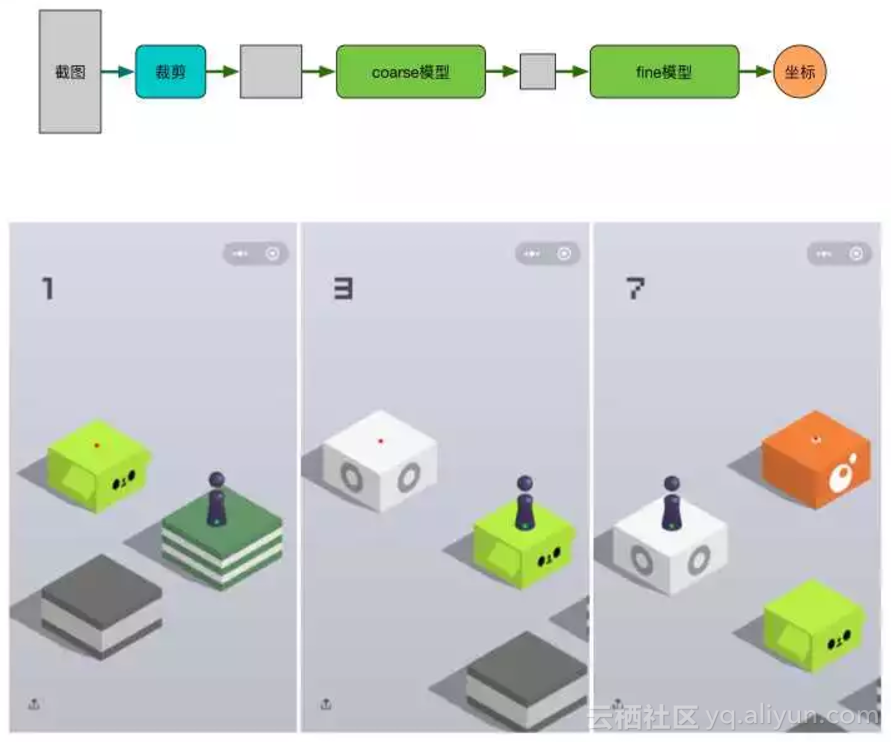2018-01-08 14:54:37

Github 地址：

https://github.com/Prinsphield/Wechat_AutoJumpdef multi_scale_search(pivot, screen, range=0.3, num=10):

H, W = screen.shape[:2]

h, w = pivot.shape[:2]

found = None

for scale in np.linspace(1-range, 1+range, num)[::-1]:

resized = cv2.resize(screen, (int(W * scale), int(H * scale)))

r = W / float(resized.shape)

if resized.shape < h or resized.shape < w:

break

res = cv2.matchTemplate(resized, pivot, cv2.TM_CCOEFF_NORMED)

loc = np.where(res >= res.max())

pos_h, pos_w = list(zip(*loc))

if found is None or res.max() > found[-1]:

found = (pos_h, pos_w, r, res.max())

if found is None: return (0,0,0,0,0)

pos_h, pos_w, r, score = found

start_h, start_w = int(pos_h * r), int(pos_w * r)

end_h, end_w = int((pos_h + h) * r), int((pos_w + w) * r)

return [start_h, start_w, end_h, end_w, score]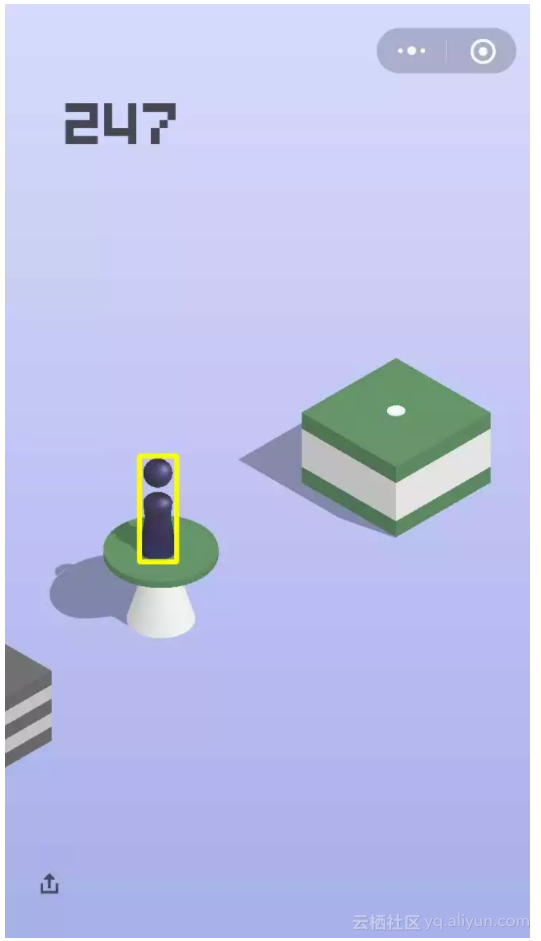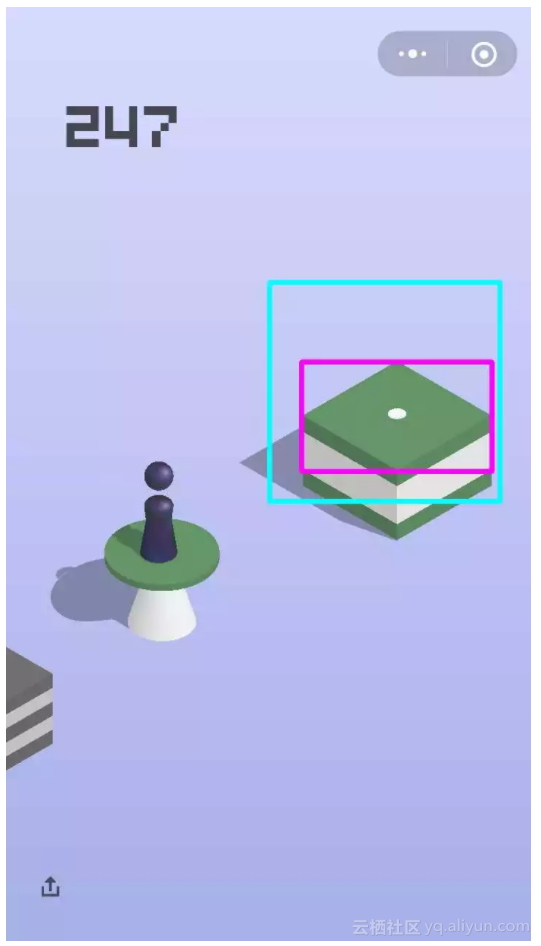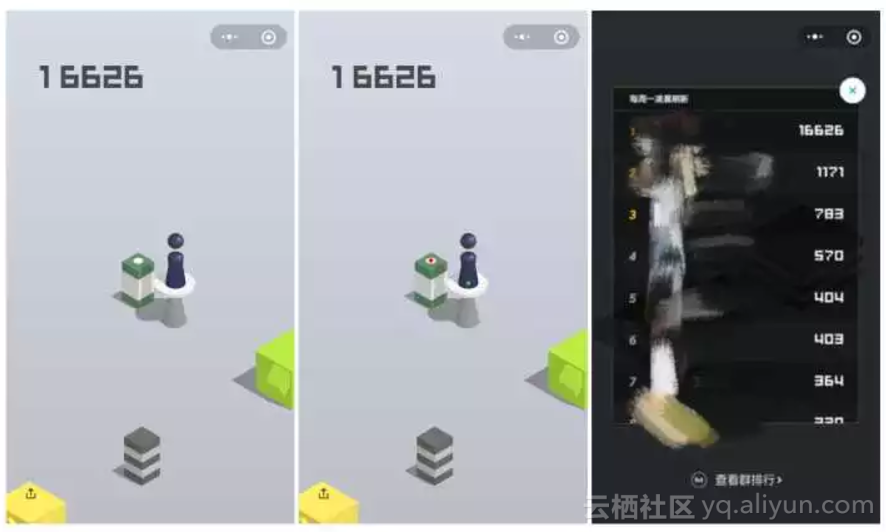CNN Coarse-to-Fine 模型

Coarse 模型数据预处理Fine 模型数据预处理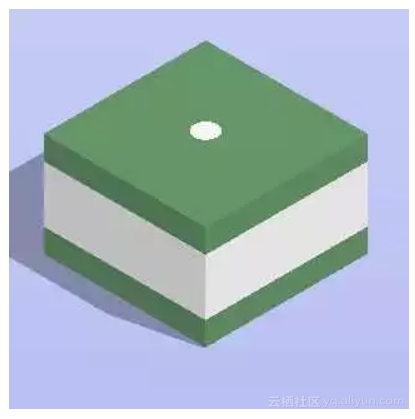Coarse 模型

def forward(self, img, is_training, keep_prob, name='coarse'):

with tf.name_scope(name):

with tf.variable_scope(name):

out = self.conv2d('conv1', img, [3, 3, self.input_channle, 16], 2)

# out = tf.layers.batch_normalization(out, name='bn1', training=is_training)

out = tf.nn.relu(out, name='relu1')

out = self.make_conv_bn_relu('conv2', out, [3, 3, 16, 32], 1, is_training)

out = tf.nn.max_pool(out, [1, 2, 2, 1], [1, 2, 2, 1], padding='SAME')

out = self.make_conv_bn_relu('conv3', out, [5, 5, 32, 64], 1, is_training)

out = tf.nn.max_pool(out, [1, 2, 2, 1], [1, 2, 2, 1], padding='SAME')

out = self.make_conv_bn_relu('conv4', out, [7, 7, 64, 128], 1, is_training)

out = tf.nn.max_pool(out, [1, 2, 2, 1], [1, 2, 2, 1], padding='SAME')

out = self.make_conv_bn_relu('conv5', out, [9, 9, 128, 256], 1, is_training)

out = tf.nn.max_pool(out, [1, 2, 2, 1], [1, 2, 2, 1], padding='SAME')

out = tf.reshape(out, [-1, 256 * 20 * 23])

out = self.make_fc('fc1', out, [256 * 20 * 23, 256], keep_prob)

out = self.make_fc('fc2', out, [256, 2], keep_prob)

return out

Fine 模型

Fine 模型结构与 coarse 模型类似，参数量稍大，fine 模型作为对 coarse 模型的 refine 操作。

def forward(self, img, is_training, keep_prob, name='fine'):

with tf.name_scope(name):

with tf.variable_scope(name):

out = self.conv2d('conv1', img, [3, 3, self.input_channle, 16], 2)

# out = tf.layers.batch_normalization(out, name='bn1', training=is_training)

out = tf.nn.relu(out, name='relu1')

out = self.make_conv_bn_relu('conv2', out, [3, 3, 16, 64], 1, is_training)

out = tf.nn.max_pool(out, [1, 2, 2, 1], [1, 2, 2, 1], padding='SAME')

out = self.make_conv_bn_relu('conv3', out, [5, 5, 64, 128], 1, is_training)

out = tf.nn.max_pool(out, [1, 2, 2, 1], [1, 2, 2, 1], padding='SAME')

out = self.make_conv_bn_relu('conv4', out, [7, 7, 128, 256], 1, is_training)

out = tf.nn.max_pool(out, [1, 2, 2, 1], [1, 2, 2, 1], padding='SAME')

out = self.make_conv_bn_relu('conv5', out, [9, 9, 256, 512], 1, is_training)

out = tf.nn.max_pool(out, [1, 2, 2, 1], [1, 2, 2, 1], padding='SAME')

out = tf.reshape(out, [-1, 512 * 10 * 10])

out = self.make_fc('fc1', out, [512 * 10 * 10, 512], keep_prob)

out = self.make_fc('fc2', out, [512, 2], keep_prob)

return out